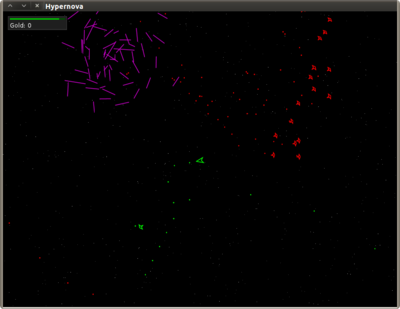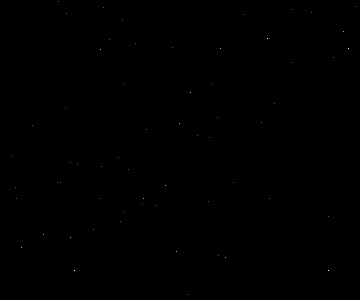## Infinite Parallax Starfield

In my free time I’ve been working on an unannounced line-art space shooter game called Hypernova. It looks a little like Asteroids, but the screen doesn’t wrap around at all. Space is infinite, and there will be all sorts of things going on out there. Quests, loot, ship upgrades and enhancements, hirelings.It will be using no bitmapped images. All the graphics are vector images described by text files. I was originally intending to follow the same path with sound effects, and rely mostly on MIDI. However, I quickly found out that many computers have no MIDI support at all.

One of the early challenges was a nice starfield background. It has several constraints:

• Can’t use any bitmapped images.
• Must work over practically infinite space.
• I must be able to resize the display
• Must be fast.

And in addition, there are some unnecessary, but desirable, properties,

• When leaving a particular location and returning, I’d like to see the same starfield pattern again.

• At the same time, I never want to see that same pattern at a different position.

When I was a kid I created the effect as a project, but it didn’t have the both the desired properties above. It’s also the method I found over and over when searching the Internet. There is an array of stars. Star positions are translated as the camera moves. If a star ever exits the display, replace it at a random position on the edge of the screen. To create a parallax effect, each star’s translation is scaled by a unique random factor.

However, there’s another algorithm I like much better, and it has both the desirable properties. Space is broken up into a grid of square tiles. To determine the star pattern in any given tile, hash the tile’s position, and use the hash output to generate a few positions within the tiles, which is where stars are drawn. To create a parallax effect, perform it in different layers at different scales.

Here’s what it looks like in the game,Hypernova is written in Java, with Clojure as a scripting language, so the starfield drawing function looks like this.

``````public static final int STAR_SEED = 0x9d2c5680;
public static final int STAR_TILE_SIZE = 256;

public void drawStars(Graphics2D g, int xoff, int yoff, int starscale) {
int size = STAR_TILE_SIZE / starscale;
int w = getWidth();
int h = getHeight();

/* Top-left tile's top-left position. */
int sx = ((xoff - w/2) / size) * size - size;
int sy = ((yoff - h/2) / size) * size - size;

/* Draw each tile currently in view. */
for (int i = sx; i <= w + sx + size * 3; i += size) {
for (int j = sy; j <= h + sy + size * 3; j += size) {
int hash = mix(STAR_SEED, i, j);
for (int n = 0; n < 3; n++) {
int px = (hash % size) + (i - xoff);
hash >>= 3;
int py = (hash % size) + (j - yoff);
hash >>= 3;
g.drawLine(px, py, px, py);
}
}
}
}
``````

Assuming the origin is in the center of the display, it iterates over each tile currently covered by the display. Positions are created by looking at the first couple bits of the hash for X, shift a few off, and looking at the first few bits again for Y. Repeat until we run out of bits. It’s called with different `starscale`s, back to front (darker to lighter), to create layers.

The `STAR_SEED` is just a Mersenne prime from the Mersenne Twister PRNG. It probably doesn’t matter much what you choose for the seed, but changing it by a single bit will drastically alter the starfield.

As far as I know, Java comes with no decent 32-bit (int) hash functions, which is really one of the biggest roadblocks in implementing effective `hashCodes()`s. Fortunately, I found an excellent hash function, Robert Jenkins’ 96 bit Mix Function, to do the trick.

``````/** Robert Jenkins' 96 bit Mix Function. */
private static int mix(int a, int b, int c) {
a=a-b;  a=a-c;  a=a^(c >>> 13);
b=b-c;  b=b-a;  b=b^(a << 8);
c=c-a;  c=c-b;  c=c^(b >>> 13);
a=a-b;  a=a-c;  a=a^(c >>> 12);
b=b-c;  b=b-a;  b=b^(a << 16);
c=c-a;  c=c-b;  c=c^(b >>> 5);
a=a-b;  a=a-c;  a=a^(c >>> 3);
b=b-c;  b=b-a;  b=b^(a << 10);
c=c-a;  c=c-b;  c=c^(b >>> 15);
return c;
}
``````

Have a comment on this article? Start a discussion in my public inbox by sending an email to ~skeeto/public-inbox@lists.sr.ht , or see existing discussions.